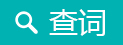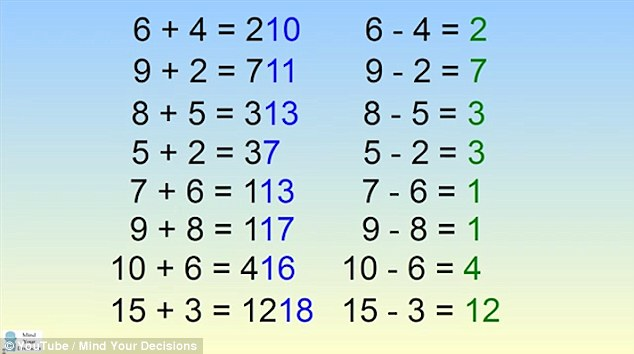# 快来测一测！会解这道题的人智商超150

Can YOU pass the intelligence test that's sweeping Facebook?

#### 中国日报网2016-05-05 10:22

A teasingly tricky maths challenge that claims to separate the real boffins from those who just claim to know the answers is sweeping Facebook.

Some three million people have already taken the tricky 'Intelligence Test' which carries a 'share if you understand' tag on it.Unsurprisingly, a shed-load of people have shared it, keen to show that they have an IQ of more than 150, apparently what you need to be able to understand it.

However, one doubting brainbox has devised a 'test for a test' to see if those who are sharing the solution to the problem really do know their stuff.

YouTuber Presh Talwalker created something which needs the same logic from the test to be applied to another piece of maths to get a correct answer.

Talwalker says of the new test: 'If you understand the rule you should be able to solve this problem.'
“Talwalker”表示：“如果你知道测试的解题规则，那么你就能答出这道题。”

Based on the intelligence test rule: x + y = (x - y) (x + y) and using the pattern below to help you, work out how ?+? = 123

6 + 4 = 210
9 + 2 = 711
8 + 5 = 313
5 + 2 = 37
7 + 6 = 113
9 + 8 = 117
10 + 6 = 416
15 + 3 = 1218If you split the answer of 123 in two: you get two numbers 1 and 23

To work back to what the numbers in question are, you need to work out two numbers where the second subtracted from the first makes 1 and also both added together makes 23.

YouTuber Talwalker called the first number in question x and the second number y.
“Talwalker”已将“?+?”中的两个数字依次命名为x和y。

Using these and the numbers 1 and 23 split out of the answer delivers:

x - y = 1
x + y = 23

This corresponds to:

12 – 11 = 1
12 + 11 = 23

Write them together and you get 123, as in the brainteaser question which is written 12 + 11 = 123x - y = 1
x + y = 23

We can add these two together, which would be written out like this (x-y)+(x+y)=24

In this equation we have 2x and no y, so:

2x=24

That means that x is 12, so using the first formula:

12-y=1

That means that y is 11.

Write these numbers in the style of the brainteaser and you get 12+11=123

Vocabulary
boffin：科学家；科技理论家
brainbox：绝顶聪明的人

### 你可能还感兴趣的文章### 中国日报网双语新闻

#### 扫描左侧二维码######Copyright by chinadaily.com.cn. All rights reserved. None of this material may be used for any commercial or public use. Reproduction in whole or in part without permission is prohibited. 版权声明：本网站所刊登的中国日报网英语点津内容，版权属中国日报网所有，未经协议授权，禁止下载使用。 欢迎愿意与本网站合作的单位或个人与我们联系。